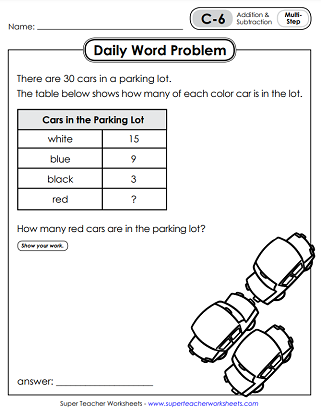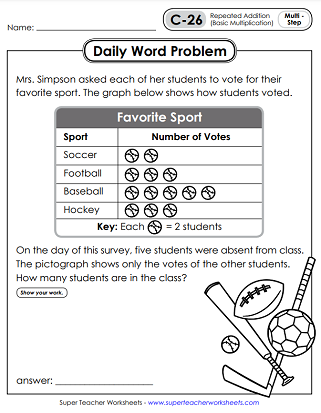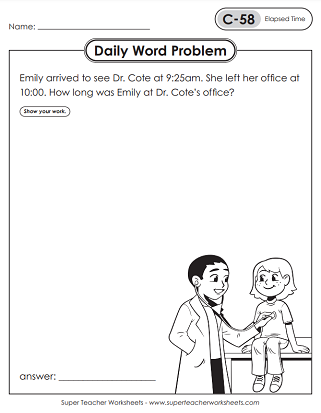# Daily Word Problems - Level C (3rd Grade)

Here you'll find Daily Math Word Problem worksheets for 3rd graders (Level C). The problems start out basic in the beginning, and become more challenging as the year goes on. Each file includes a mix of one-step and multi-step problems.This file contains 5 worksheets, with one word problem on each page. There are 3 multi-step problems and 2 one-step problems.
(Click the 'Alt' button below if you prefer color poster versions of these problems.)
This file has five more word problems- one for each day of the school week. In this set, students will use addition, subtraction, and basic multiplication (repeated addition) to solve single and multi-step problems.
Students will use math skills to answer a series of questions about golf balls, coins, t-shirts, and crayons.
This set has multiple-step problems with addition and subtraction, as well as single-step multiplication problem, and a special place value challenge.
In this file, you'll see a new 3rd grade word problem for each day of the week. Skills include counting money, addition, and subtraction, as well as basic multiplication.
In these single-step and multi-step problems, your students will review repeated addition (basic multiplication), as well as addition and subtraction.
This file includes a multi-step problem that requires both subtraction and multiplication. There is also a problem where students must draw an array to multiply.
Skills on this set of worksheets includes: reading and interpreting a line plot; solving a basic multiplication word problem; solving a basic division problem; and completing a multi-step problem involving multiplication and addition.
Review elapsed time, fractions of a set, basic multiplication & division, and reading data on a line plot.
Solve multi-step word problems using knowledge of the four operations. Compare fractions of pizzas. Solve a basic multiplication and division problem.
Determine how much liquid is in each of the two beakers and subtract to find the difference. Solve multi-step problems using knowledge of the four operations. Divide \$20 equally among four children.
Interpret data on a table. Review multi-digit addition and subtraction. Calculate elapsed time. Divide 18 items equally among three people.
This set of five daily word problems includes multi-step operations problems, elapsed time, and more!
This next set of daily word problems includes several multi-step operations problems and an elapsed time problem.
This set of daily word problems includes deciphering a graph, interpreting data on a table, and operations problems.
Operations with money, measurement, and elapsed time are some of the skills students will work on with this week's daily math problems.
Math Buzz

Math Buzz is a daily math review series. There are 150 spiraling review worksheets in the set. Math Buzz covers a wide range of age-appropriate of skills, including time, money, place value, operations, geometry, area, perimeter, measurement, and fractions.

More Daily Word Problems

STW has daily word problems for other grades as well. Check them out! All done with the 3rd grade Daily Word Problems? Then move ahead to 4th!

## Sample Worksheet ImagesMy Account
Site Information